Math resources Geometry Perimeter

Perimeter of a triangle

# Perimeter of a triangle

Here you will learn how to find the perimeter of a triangle, including what the perimeter is, how to calculate it and how to solve perimeter word problems.

Students will first learn how to find perimeter as part of measurement and data in 3rd grade.

## What is the perimeter of a triangle?

The perimeter of a triangle is the total distance around the outside of the triangle.

For example,

Perimeter is measured in units, like inches.

Measuring the perimeter is like starting at one vertex of a triangle and measuring the total distance around the triangle.

The perimeter can also be calculated by adding the length of each side.

7 + 7 + 3 = 17, so the perimeter is 17 inches.

### What is the perimeter of a triangle?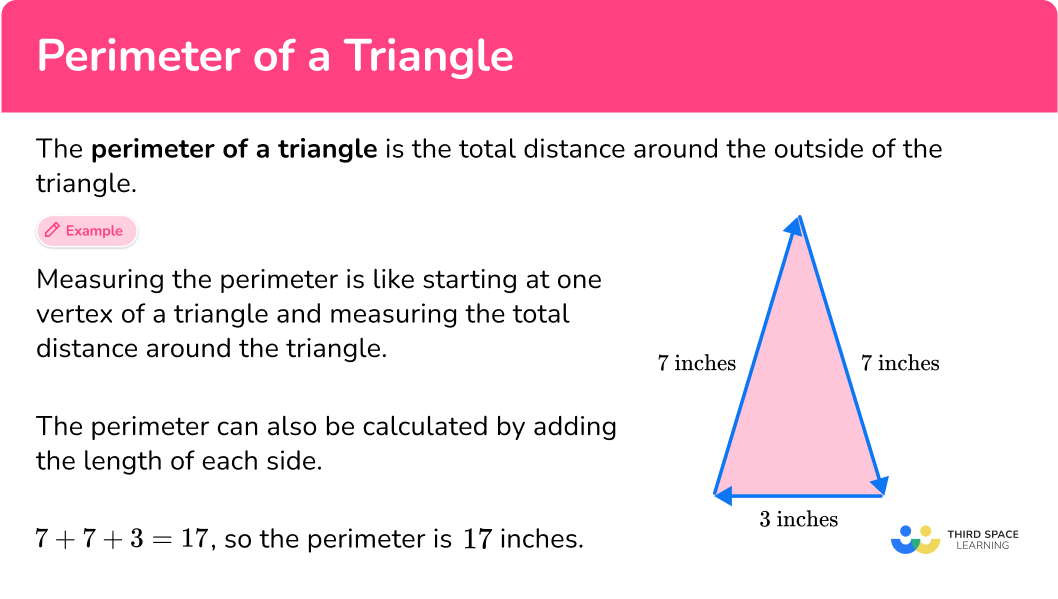## Common Core State Standards

How does this relate to 3rd grade math?

• Grade 3 – Measurement and data (3.MD.D.8)
Solve real world and mathematical problems involving perimeters of polygons, including finding the perimeter given the side lengths, finding an unknown side length, and exhibiting rectangles with the same perimeter and different areas or with the same area and different perimeters.

## How to find the perimeter of a triangle

In order to calculate the perimeter of a triangle:

1. Add all the side lengths.
2. Write the final answer with the correct units.

## How to find perimeter examples

### Example 1: perimeter of an isosceles triangle

What is the perimeter of the triangle?

1. Add all the side lengths.

To find the perimeter (the total distance around the triangle), add all the side lengths:

9 + 9 + 12 = 30

2Write the final answer with the correct units.

The side lengths are measured in feet, so the total perimeter is in feet.

The perimeter of the triangle is 30 feet.

### Example 2: perimeter of a right triangle

What is the perimeter of the triangle?

To find the perimeter (the total distance around the triangle), add all the side lengths:

12 + 20 + 16 = 48

The side lengths are measured in millimeters, so the total perimeter is in millimeters.

The perimeter of the triangle is 48 millimeters.

### Example 3: perimeter of a scalene triangle

What is the perimeter of the triangle?

To find the perimeter (the total distance around the triangle), add all the side lengths:

11 + 17 + 8 = 36

The side lengths are measured in centimeters, so the total perimeter is in centimeters.

The perimeter of the triangle is 36 centimeters.

### Example 4: perimeter of equilateral triangle

What is the perimeter of the triangle?

To find the perimeter (the total distance around the triangle), add all the equal side lengths:

16 + 16 + 16 = 48

For the perimeter of an equilateral triangle, since the sides of the triangle are the same (congruent), you can also multiply one side length by 3.

3 \times 16 = 48

The side lengths are measured in meters, so the total perimeter is in meters.

The perimeter of the triangle is 48 meters.

### Example 5: triangle perimeter word problem

A triangular street sign has side lengths of 18 inches, 18 inches and 16 inches. What is the perimeter of the sign?

To find the perimeter (the total distance around the triangle), add all the side lengths:

18 + 18 + 16 = 52

The side lengths are measured in inches, so the total perimeter is in inches.

The perimeter of the sign is 52 inches.

### Example 6: right scalene triangle

The perimeter of the triangle is 75{~cm}. What is the missing side length?

To find the perimeter (the total distance around the triangle), add all the side lengths:

\begin{aligned} & 17 \, + \, 31 \, + \,? = 75 \\\\ & 48 \, + \, ? = 75 \end{aligned}

The two sides together are 48. The third side is missing.

To find the missing side, think about what number added to 48 is 75.

Since 48 + 27 = 75, the missing side length is 27.

The side lengths are measured in centimeters and the total perimeter is in centimeters.

The missing side length of the triangle is 27 centimeters.

### Teaching tips for the perimeter of a triangle

• Choose worksheets that have a variety of question types – varying the types of triangles – equilateral, isosceles and scalene (therefore varying the lengths of the sides), solving for the perimeter or a missing side length, word problems with or without visuals.

• Give students opportunities to measure and solve problems for the perimeter of triangles in the real world.

### Easy mistakes to make

• Thinking the order of adding the sides matters
It doesn’t matter the order in which the sides of the triangle are added, because of the commutative property of addition. As long as all sides are added only once, they can be added in any order.

• Confusing the formulas or solving strategies for perimeter with area
It is easy to confuse these two concepts, especially when first learning them. Remember that perimeter is the measurement of the length around a triangle (one-dimensional), and area is the measurement of the space within a triangle (two-dimensional).

Side lengths need to be the same units before they can be added. If the sides are shown with different units, convert them to a common unit and then add.

### Practice perimeter of a triangle questions

1) What is the perimeter of the triangle?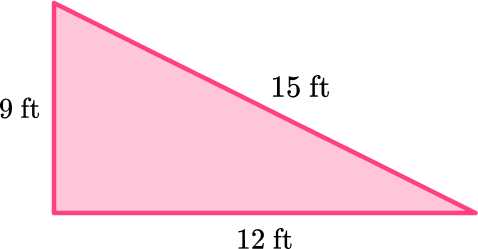36{~ft}32{~ft}26{~ft}54{~ft}The perimeter (the total distance around the triangle) is the sum of the lengths of the three sides:

9 + 15 + 12 = 36

The sides are measured in feet, so the perimeter is also in feet.

The perimeter is 36 feet.

2) What is the perimeter of triangle ABC?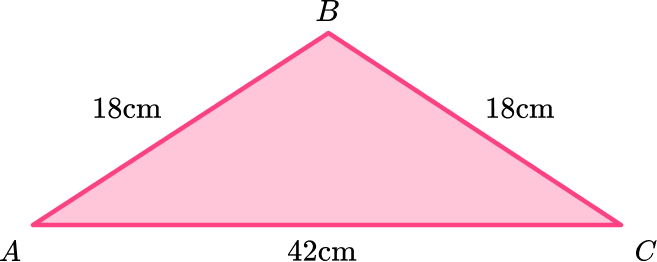18{~cm}84{~cm}78{~cm}68{~cm}The perimeter (the total distance around the triangle) is the sum of the lengths of the three sides:

18 + 18 + 42 = 78

The sides are measured in centimeters, so the perimeter is also in centimeters.

The perimeter of triangle ABC is 78{~cm}.

3) Which triangle has a perimeter of 41 units?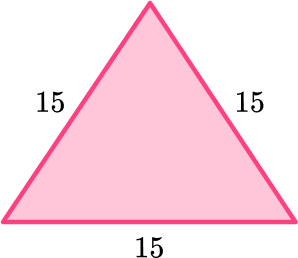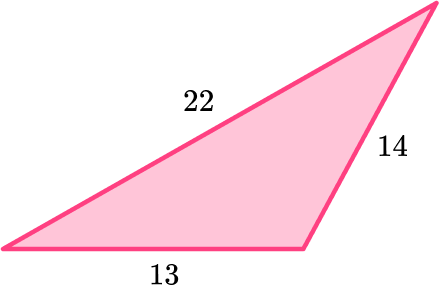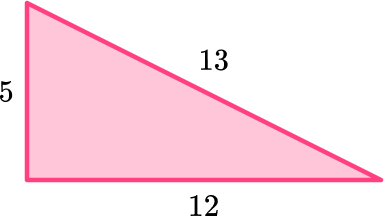Since the perimeter of the triangle is 41 units, the length of its sides should add up to 41.17 + 17 + 7 = 41

This triangle has a perimeter of 41 units.

4) Which are the sides of a triangle with a perimeter of 50{~cm}?

three sides of 20{~cm}12{~cm}, \, 13 {~cm}, \, 14{~cm}15{~cm}, \, 20{~cm}, \, 15{~cm}20{~cm}, \, 5{~cm}, \, 20{~cm}, \, 5{~cm}A triangle has 3 sides. The perimeter of a triangle is the sum of the 3 side lengths:

15 + 20 + 15 = 50

The sides of a triangle 15{~cm}, 20{~cm}, and 15{~cm} have a perimeter of 50{~cm}.

5) The perimeter of the triangle is 67 inches. What is the length of the third side?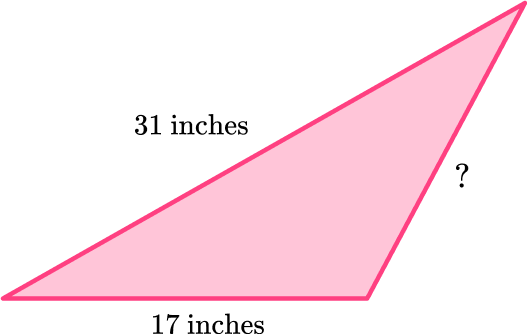17 inches22 inches15 inches19 inchesThe perimeter (the total distance around the triangle) is the sum of the sides:

\begin{aligned} & 31 \, + \, 17 \, + \, ? = 67 \\\\ & 48 \, + \, ? = 67 \end{aligned}

The two sides together are 48. The third side is missing.

To find the missing side, think about what number added to 48 is 67.

Since 48 + 19 = 67, the missing side length is 19 inches.

6) The side of a large garden, shaped like an equilateral triangle, is 23 meters. The side of a smaller garden, shaped like an equilateral triangle, is 18 meters. What is the difference in the perimeter of the two gardens?

5 meters13 meters41 meters15 metersEach side of an equilateral triangle is the same length, so all sides of the large garden are 23 meters and all sides of the smaller garden are 18 meters.

Large Garden: Small Garden: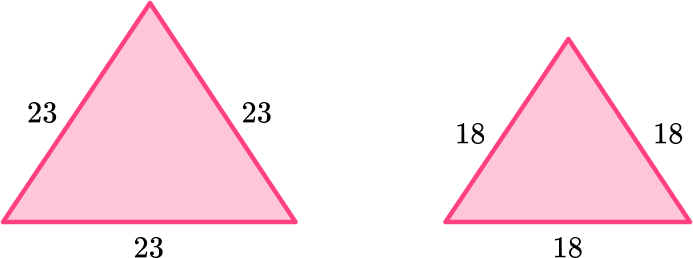Perimeter: Perimeter:

23+23+23=69 meters \hspace{1.1cm} 18+18+18=54 meters

To find the difference between the perimeter of the large and small garden, subtract:

69 \, – \, 54 = 15

The difference in perimeter is 15 meters.

## Perimeter of a triangle FAQs

How do you calculate the perimeter of different types of triangles?

Since all triangles have 3 sides, just add the length of all three sides to find the perimeter. There are additional ways to solve for different types of triangles, like equilateral and isosceles triangles.

For equilateral triangles, multiply the length of one side by 3. For isosceles triangles, multiply one of the equal sides by 2 and then add the third side.

How do you calculate the area of a triangle?

The area of a triangle can be calculated with the formula: \cfrac{1}{2} \times b \times h.

Can the given sides of a triangle be fractions or decimals?

Yes, triangles can have measurements that include fractions or decimals. The perimeter is found the same way – by adding all the sides.

What is the pythagorean theorem?

It is a theorem that relates to right-angled triangles and the relationship between the two sides that form a 90 -degree angle and the long side, called the hypotenuse. This is a topic covered in middle school.

## Still stuck?

At Third Space Learning, we specialize in helping teachers and school leaders to provide personalized math support for more of their students through high-quality, online one-on-one math tutoring delivered by subject experts.

Each week, our tutors support thousands of students who are at risk of not meeting their grade-level expectations, and help accelerate their progress and boost their confidence.